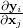# RTTOV – Mathematical Overview

## Mathematical overview of RTTOV

Given a state vector, x, which describes the atmospheric/surface state and a radiance vector, y, then for all channels required to be simulated:

y = H(x)

where H is the radiative transfer model, i.e. RTTOV (also referred to as the observation operator in data assimilation parlance). This is known as the ‘direct’ or ‘forward’ model.

In addition RTTOV also computes the Jacobian matrix H which gives the change in radiance δy for a change in any element of the state vector δx assuming a linear relationship about a given atmospheric state x0:

δy = H(x0)δx

The elements, Hi,j, of H contain the partial derivativeswhere the subscript i refers to channel number and j to position in state vector. The Jacobian gives the top of atmosphere radiance change for each channel from each level in the profile given a unit perturbation at any level of the profile vectors or in any of the surface/cloud parameters. It shows clearly, for a given profile, which levels in the atmosphere are most sensitive to changes in temperature and variable gas concentrations for each channel. RTTOV_K (and its associated subroutines ending in K) compute the H(x0) matrix for each input profile.

It is not always necessary to store and access the full Jacobian matrix H and so the RTTOV package also has routines to only output the tangent linear values δy, i.e. the change in top of atmosphere radiances, for a given change in atmospheric profile, δx, about an initial atmospheric state x0. The tangent linear routines all have TL as an ending. Conversely the adjoint routines (ending in AD) compute the change in the gradient of any scalar quantity with respect to the atmospheric state, x0, given a change in the gradient of that quantity with respect to the radiances, y. These routines are normally used as part of the variational assimilation of radiances.

For users only interested in the direct or forward model for radiance simulations the TL/AD/K routines are not required.